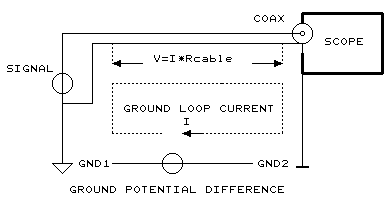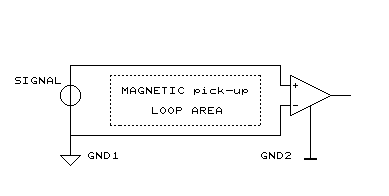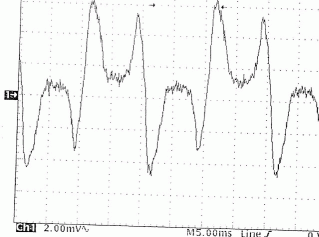QT designed instrumentation -measure-loops

 A ground-loop itself is actually not a reason for interference. What causes the problem is the fact that a ground-line is also used as a zero-reference (zero-loop) .Power-line grounds carry large displacement currents, if they run through the zero reference-line (being non zero-ohm) this signal adds up to the measurement signal Solution: use differential inputsThis is what the result of a ground-loop looks on an oscilloscope:Even when using differential inputs, there is a coupling of magnetic flux from power-transformers possible in the loop area of the (shielded) wires solutions: minimize loop-area, move away from tranformersThis is what the result of a magnetic-pickup-loop looks on an oscilloscope: